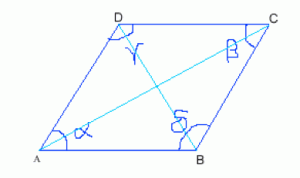#Difference Between Similar Terms and Objects

# Difference Between Rhombus and Parallelogram

Rhombus vs Parallelogram

A parallelogram is a quadrilateral or four-sided figure in which the opposite sides are parallel. And because the opposite lines are parallel, hence the opposite angles are also equal.

A rhombus, on the other hand, may be defined as an equilateral parallelogram. It is a four-sided figure which has all the four sides equal. This property of a rhombus is similar to a square. The distinguishing characteristic between the two is that a square has all the angles equal to 90 degrees, but in a rhombus only the opposite angles are equal. Still, there is a distinguishing feature that in a rectangle the opposite angle is equal to 90 degrees, but in the case of a rhombus, the angles are not equal to 90 degrees. They are supplementary angles.
Thus we may say that every rhombus is a parallelogram but the converse is not true.

A rhombus is also called as a diamond or a lozenge.

We will take the help of the above figure to discuss a parallelogram and a rhombus one by one.Rhombus
In a rhombus:
The angle α=angle β and angle δ=angle γ. But angle α=angle β is unequal to angle δ=angle γ.
The diagonals AC and BD intersect each other making a right angle (a right angle is an angle of 90 degrees) or are parallel to each other.
The diagonals bisect the opposite angles.
The circumference or perimeter of a rhombus may be calculated as:

Circumference = 4 x side.

Parallelogram
In a parallelogram:
The opposite sides are equal i.e., AB=CD and BC=AD.
The angle α=angle β and angle δ=angle γ
The angle may be equal to 90 degrees. (This is the case of a rectangle.)
Each of the diagonals forms a triangle which is congruent to each other.
The diagonals bisect the opposite angles.
The circumference or perimeter of a parallelogram may be calculated as:

Circumference = 2 (AB + BC).

Summary:
In a parallelogram, the opposite sides are equal whereas in a rhombus all four sides are equal.
In a parallelogram, the diagonals bisect each other whereas in a rhombus they do not bisect each other.
In a rhombus, the diagonals intersect each other at right angles and hence are perpendicular to each other. It is not so in the case of a parallelogram.
In a parallelogram, the angles may be equal to 90 degrees, but it can never be 90 degrees in the case of a rhombus.
A rhombus may be regarded as a subset of a parallelogram.

Latest posts by Lathan K (see all)

### Search DifferenceBetween.net :

Custom Search

Help us improve. Rate this post!(7 votes, average: 3.57 out of 5)Loading...Email This Post : If you like this article or our site. Please spread the word. Share it with your friends/family.

1. Good